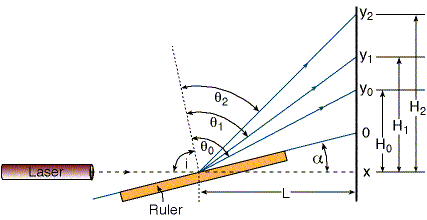Rami Arieli: "The Laser Adventure", Ruler Lab., Page 1
Interference experiment using a Ruler

This experiment was first demonstrated by A. L. Schawlow(1).
In this experiment you measure the laser wavelength with a simple ruler (!)
Schawlow is the scientist who received the Nobel price for the invention of the idea of the laser.

Objective: To calculate the wavelength of laser light using a simple ruler as a diffraction grating.

Theory: In this interference experiment, a simple ruler will serve as a grating.

The experimental set up is shown in Figure 1:Figure 1: The experimental setup.
Point x is the original mark of the laser beam (without the ruler).
Point y0 is the first point of light on the screen.
Point O is half the distance between point x and y0. This point is the origin for the measurements (The distances y0, y1, y2, ..., yn are measured from this point).
For small angles (a), point O is approximately the point showing the direction of the ruler.

i = Angle between the laser beam and the perpendicular to the ruler.

qm = Angle between the perpendicular to the ruler and the reflected beam.

d = Smallest distance between neighboring marks on the ruler.

L = Distance from the ruler (where the laser beam hits) to the screen.

a = Grazing angle between the ruler and the original direction of the laser beam (very small angle, between 2-60).